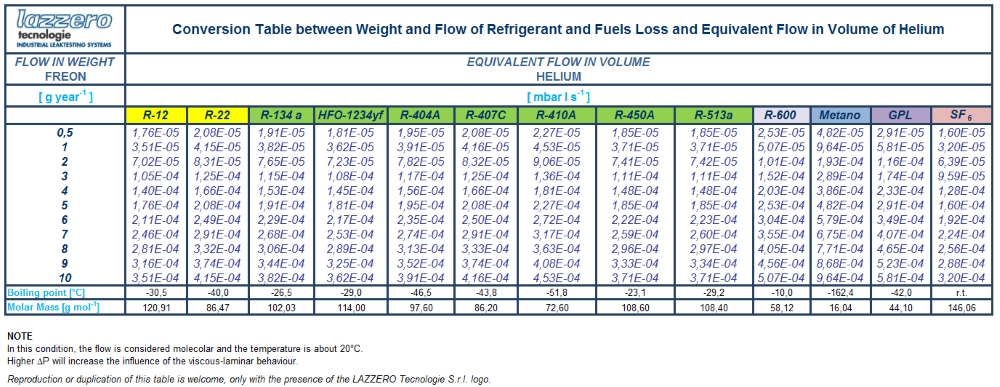A leak may be considered a ‘chink’ through which gas leaks, in a system understood to be hermetic. In terms of dimensions, a leak is quantified by indication of the quantity of gas (expressed in volume) leaking, with a given ΔP at the sides of the leak, in one second. A gas flow takes place in a channel if, between the channel’s extremities, a pressure difference is maintained. The capacity of a channel to enable passage of the gas flow depends not only on the pressure difference between the two extremities of the channel but also on the channel’s geometric characteristics. A magnitude (conductance) is therefore adopted. This is defined as the relation between the gas flow and the pressure difference between the extremities of the channel: C = Q / (p1 - p2) expressed as m3/s or l/s.

• The ideal gases equation finds expression in the following formula:where n is the number of moles of gas contained in volume V at pressure p and absolute temperature (Kelvin degrees [K]), and R is the gases constant (8.31441 j·mol-1·K-1). In this manner, if the pressure is a constant of the system, the equation is obtained, with the flow dependent upon gas volume variation, on pressure and on time:The unit of measurement for the leakrate (Q) according to the International System (SI) is Pa·m3/s. The mbar·l/s unit is a permissible unit of measurement of flow, according to the SI, since it is linked to the former unit of measurement by multiples.

1 mbar·l/s = 10-1 Pa·m3/s (1 Pa = 10-2 mbar and 1 m3 = 103 litres).

In the case of mbarL/s units, the leakrate is Q= 1 mbar·l·s-1 if, in a closed and evacuated chamber of a volume of 1 litre, the pressure increases, in 1 second, by 1 mbar, or, in the case of chamber overpressure, decreases by 1 mbar.

However, it is not always possible to have the leak value expressed in international system units of measurement. It is therefore appropriate to convert these values into Pa·m3/s or mbar·l/s.
A specific instance is to be found in the coolants sector, where coolant leak values are expressed as g/year. Mass flow in this case is transformed into g/Mole and then Moles/s so it is finally transformed into gaseous flow. A table is provided below in which conversion from mass flow to equivalent flow is applied, specifying conversion of g/year values of the key coolants and other gases into the equivalent nominal flows of helium in mbar·l/s.The transformation from the stream by weight (g / year) equivalent flow of helium (mbar·l/s) can be simply achieved by multiplying g / year for the following factors:By using this site, you agree that we and our partners may set cookies to personalize content according to our Cookies Policy. If you want to know more, click on "More informations" if you do not agree please close this browser window.